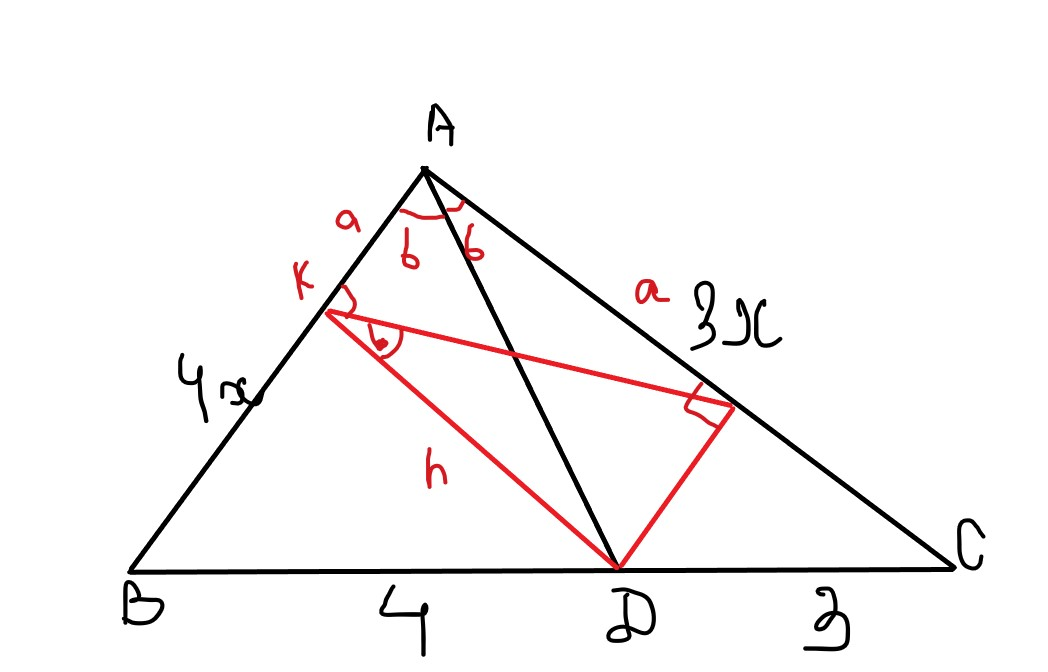Deromediqm

2022-07-23

In $\mathrm{△}ABC$, let D be a point on BC such that AD bisects $\mathrm{\angle }A$. If $AD=6$, $BD=4$ and $DC=3$, then find AB.edgarovhg

Expert

Step 1
Let E is point of line AD such that $\mathrm{\angle }AEB=90°$ and F is point of line AD such that $\mathrm{\angle }AFC=90°$. Then triangles ABE and ACF are similar, triangles DBE and DCF are similar. Using this facts, one can write $\frac{AF}{AE}=\frac{CF}{BE}=\frac{CD}{BD}=\frac{3}{4}$
$\frac{DF}{DE}=\frac{CD}{BD}=\frac{3}{4}$
Step 2
Then $\frac{EF}{AE}=1-\frac{AF}{AE}=\frac{1}{4}$
$\frac{EF}{DE}=1+\frac{DF}{DE}=\frac{7}{4}$
$\frac{AE}{DE}=\frac{EF/DE}{EF/AE}=7$
$\frac{AD}{DE}=\frac{AE}{DE}-1=6$
$DE=AD/6=1$
$AE=7\cdot DE=7$
$B{E}^{2}=B{D}^{2}-D{E}^{2}=16-1=15$
$A{B}^{2}=A{E}^{2}+B{E}^{2}=49+15=64$
$AB=8$equissupnica7

Expert

Step 1With angle bisector theorem you can easily find out that $\frac{AB}{AC}=\frac{4}{3}$. By this relationship, you can label sides $AB=4x,AC=3x$.
Draw perpendicular DK, DL such that points D, L lies on the sides AB, AC respectively.
You can easily find out that $\mathrm{△}AKD\cong \mathrm{△}DLA$ (A. A. S.)
By this, you can label $KD=DL=h$ and $AK=AL=a$
Step 2
By the Pythagorean theorem, you can get the following equations,
${h}^{2}+{a}^{2}=36$ (1)
${h}^{2}+\left(3x-a{\right)}^{2}=9$ (2)
${h}^{2}+\left(4x-a{\right)}^{2}=16$ (3)
Expanding (2), (3) and substituting (1) as required will lead you to $x=2$
As $AB=4x,AB=2\ast 4=8$

Do you have a similar question?